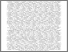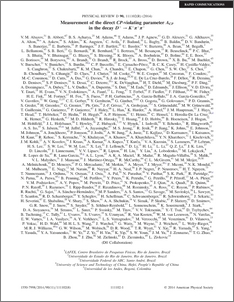Measurement of the direct CP-violating parameter A_CP in the decay D+ -> K- pi+ pi+

, D0 Collaboration (2014) Measurement of the direct CP-violating parameter A_CP in the decay D+ -> K- pi+ pi+. Physical Review D, 90 (11). ISSN 1550-7998Preview
PDF (PhysRevD.90.111102)
PhysRevD.90.111102.pdf - Published Version

Abstract

We measure the direct CP-violating parameter A_CP for the decay of the charged charm meson, D+ -> K-pi+pi+ (and charge conjugate), using the full 10.4 fb-1 sample of ppbar collisions at sqrt(s) = 1.96 TeV collected by the D0 detector at the Fermilab Tevatron collider. We extract the raw reconstructed charge asymmetry by fitting the invariant mass distributions for the sum and difference of charge-specific samples. This quantity is then corrected for detector-related asymmetries using data-driven methods and for possible physics asymmetries (from B -> D processes) using input from Monte Carlo simulation. We measure A_CP = [-0.16 +- 0.15 (stat.) +- 0.09 (syst.)]%, which is consistent with zero, as expected from the standard model prediction of CP conservation, and is the most precise measurement of this quantity to date

Item Type:
Journal Article
Journal or Publication Title:
Physical Review D
Subjects:
Departments:
ID Code:
71737
Deposited By:
Deposited On:
13 Nov 2014 11:18
Refereed?:
Yes
Published?:
Published